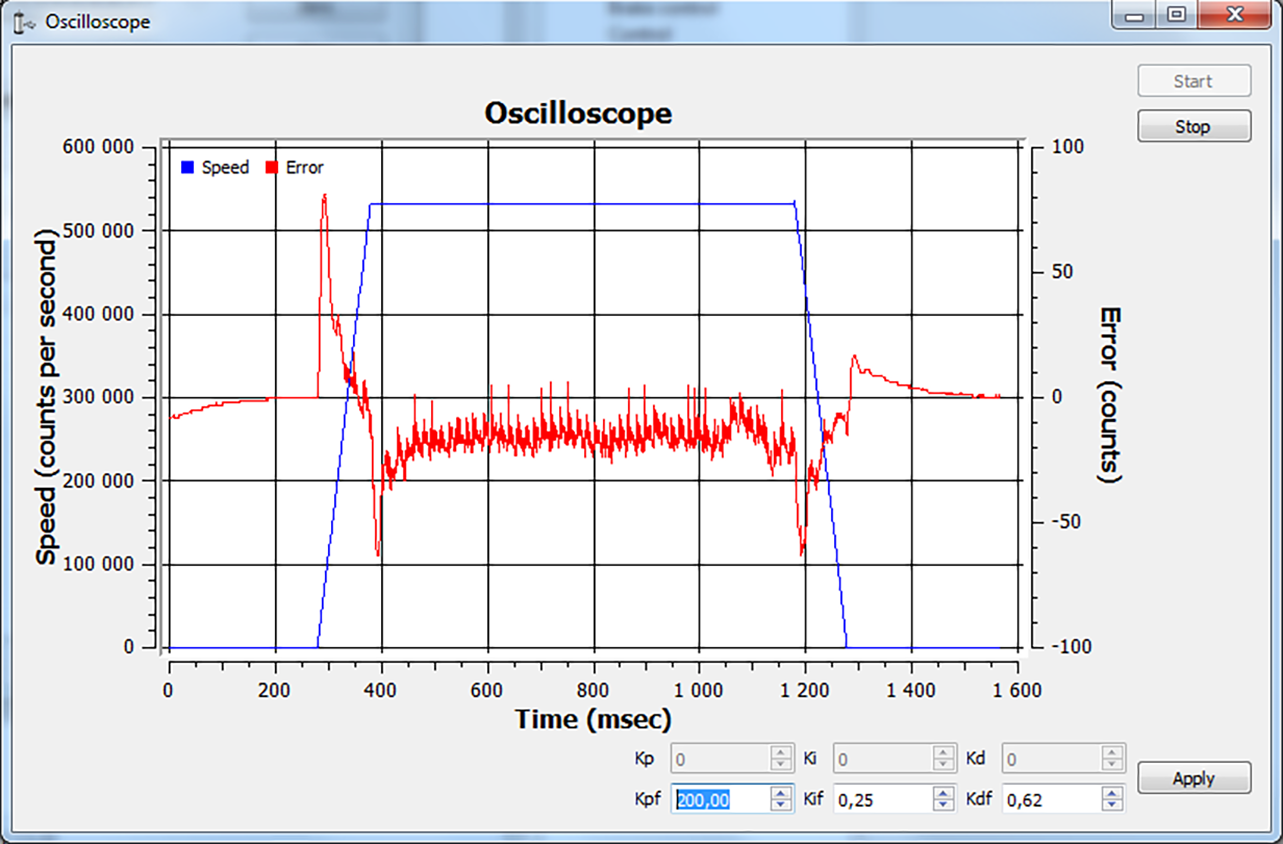# 4.2.13. PID-algorithm for BLDC engine control¶

## 4.2.13.1. PID-algorithm description¶

BLDC engine is controlled by the PID regulator, with the coordinate as the controlled parameter. The controlled coordinate changes according to motion settings and incoming commands to provide motion capability. We will call controller coordinate the running position. Output current is the control signal of the regulator. The control action is calculated according to the following formula:

$U(t) = I + P + D = K_p \cdot E(t) + K_i\int E(t)dt + K_d\frac{dE(t)}{dt}, where:$

$$U(t)$$ - is the control action

$$E(t)$$ - is difference between the running coordinate and the current motor coordinate

$$K_p, K_i, K_d$$ - are proportional, integral and differential coefficients of the regulator. Regulator coefficients are set on PID settings page of the XiLab program or programmatically by calling set_pid_settings() function of the libximc library (see Programming guide).

The effects different PID components (Kp, Ki, Kd) have are same for BLDC and DC motors. See PID-algorithm for dc engine control.

## 4.2.13.2. PID regulator manual tuning¶

We provide a special XiLab extension for the manual adjustment of the PID regulator coefficients. The time dependence of the speed of the BLDC engine and the speed retention error is shown in a special window, see the screenshot below.The PID regulator tuning window.

The stable position retention is necessary for the correct engine operation.

### 4.2.13.2.1. Steps to adjust the coefficients:¶

1. First, you need to evaluate the PID coefficients. Given the structure of the managed system, they can be calculated from simplified formulas. For this, the parameters from the documentation for the appropriate motor and stage are used.
• $$K_m$$ - electromechanical motor coefficient [H / A] (the torque generated by the current strength is 1 A). Can be calculated as the ratio $$K_m = \frac{F_n}{I_n}$$, where $$F_n$$ is the nominal (maximum) force generated by the motor, $$I_n$$ is the rated (maximum) current strength.
• $$M$$ - weight of load (kg).
• $$\sigma = \frac{M}{K_m}$$.
• $$K_p = 11500 \sigma \cdot 1000, \; K_d = 186 \sigma \cdot 1000, \; K_d = 12.2 \sigma \cdot 1000.$$
2. Set the coefficients calculated by formulas, click Apply. Click the Zero button on the main XiLab window. Set 0 to the Move to field, send the command. The engine should stop. Try to move the position manually, make sure that the response is correct - the engine tries to return to zero position (the encoder reverse is set correctly).
3. Set a small speed in the motion settings, click Apply. Start moving in the main window. The differential coefficient (Kdf) should be increased if there are vibrations and disruptions.
4. If the vibrations have audio frequencies (the stage emits a loud sound when driving), it may be necessary to reduce the Kd coefficient or all the coefficients proportionally.
5. The integral coefficient (Kif) is responsible for getting into the target position, it is convenient to use the command Shift on for testing.
6. To fine-tune the coefficients use the Oscilloscope window where the speed retention error is displayed for used motion parameters.

Note

Only special XiLab version support oscilloscope window. Contact us to get it.

1. After the coefficients are adjusted, they need to be proportionally increased/decreased, this corresponds to an increase/decrease in mass, response to the impact becomes more/less powerful, sudden stops will not lead to disruption of movement.